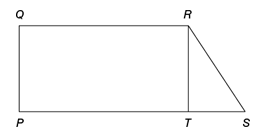Payal Tandon
Co-founder, e-GMAT
Welcome to e-GMAT Support!
I am Payal, Co-Founder of e-GMAT.
Feel free to ask any Query.We will be contacting you soon on

## OG 2020: Question No. 335In the figure above, PQRT is a rectangle. What is the length of segment PQ?

1. The area of region PQRS is 39 and TS = 6.
2. The area of region PQRT is 30 and QR = 10.
 Source OG 2020 Type Data Sufficiency Topic Geometry Sub-Topic Quadrilateral Difficulty Medium

### Solution

#### Steps 1 & 2: Understand Question and Draw Inferences

In this question, we are given

• A diagram of a quadrilateral PQRS, of which PQRT is a rectangle

We need to determine

• The length of segment PQ

Since PQRT is a rectangle we can say the side QR and PT are parallel to each other.

• Therefore, we can say that PQRS is a trapezium.

With this understanding, let us know analyse the individual statements.

#### Step 3: Analyse Statement 1

As per the information given in statement 1, the area of region PQRS is 39 and TS is equal to 6.

• Area of PQRS = (1/2) (QR + PS) (PQ) = 39

Or, (QR + PS) (PQ) = 78

Or, (PT + PT + TS) (PQ) = 78

Or, (2PT + 6) (PQ) = 78

As we don’t know the value of PT, we cannot determine the value of PQ.

Hence, statement 1 is not sufficient to answer the question.

#### Step 4: Analyse Statement 2

As per the information given in statement 2, the area of region PQRT is 30 and QR is equal to 10.

• PQ * QR = 30

Or, PQ * 10 = 30

Or, PQ = 3

As we can determine the value of PQ, statement 2 is sufficient to answer the question.

#### Step 5: Combine Both Statements Together (If Needed)

Since we can determine the answer from statement 2 individually, this step is not required.

Hence, the correct answer choice is option B.

Questions on Geometry are very commonly asked on the GMAT. Ace GMAT Quant by signing up for our free trial and get access to 400+ questions. We are the most reviewed online GMAT Prep company with 2400+ reviews on GMATClub.#### Juhi Gupta

###### Browse Related Topics# Achieve 740+on the GMAT in 30 days!